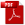Download as PDFAsymptotic formulæ in combinatory analysis
Proceedings of the London Mathematical Society, 2, XVI, 1917, Records for 1 March 1917

A preliminary account of some of the contents of this paper1 appeared in the Comptes Rendus of January 2nd, 1917. The paper contains a full discussion and proof of the results there stated. The asymptotic formula for $p(n)$, the number of unrestricted partitions of $n$, of which only the first three terms were given, is completed; and it is shewn that, by taking a number of terms of order $\sqrt{n}$, the exact value of $p(n)$ can be obtained for all sufficiently large values of $n$. Some account is also given of actual or possible applications of the method used to other problems in Combinatory Analysis or the Analytic Theory of Numbers.

Endnotes

1[No. 36 of this volume.]